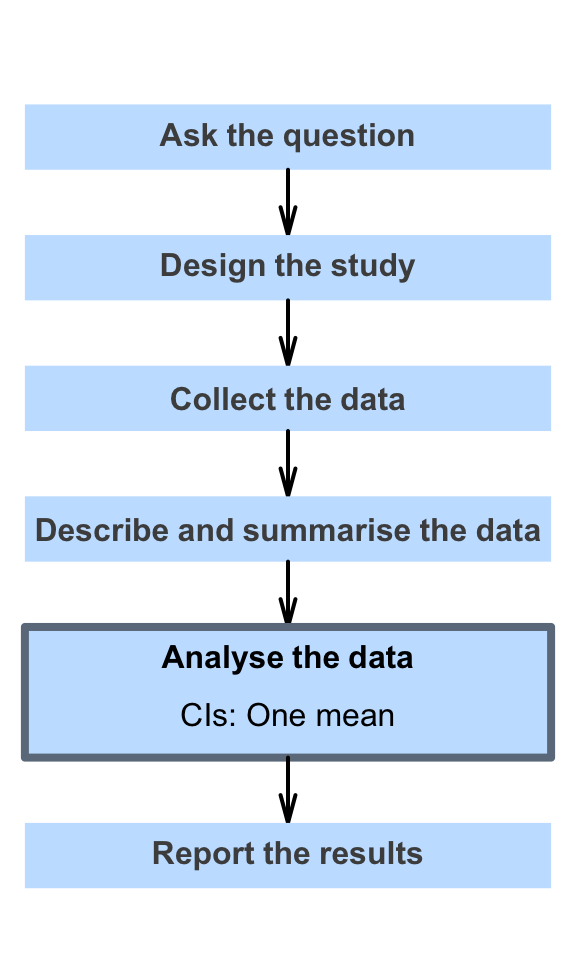# 22 Confidence intervals for one mean

So far, you have learnt to ask a RQ, identify different ways of obtaining data, design the study, collect the data describe the data, summarise data graphically and numerically, and understand the tools of inference.

In this chapter, you will learn about confidence intervals for one mean. You will learn to:

• produce confidence intervals for one mean.
• determine whether the conditions for using the confidence intervals apply in a given situation.
• compute sample size estimates in this situation.